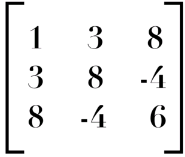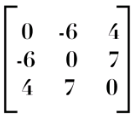Courses

# Matrices Part 2 Mathematics Notes | EduRev

## Algebra for IIT JAM Mathematics

Created by: Veda Institute

## Mathematics : Matrices Part 2 Mathematics Notes | EduRev

The document Matrices Part 2 Mathematics Notes | EduRev is a part of the Mathematics Course Algebra for IIT JAM Mathematics.
All you need of Mathematics at this link: Mathematics

Symmetric and Skew-Symmetric Matrices
As we are all aware by now that equal matrices have equal dimensions, hence only the square matrices can be symmetric or skew-symmetric form. Now, what is a symmetric matrix and a skew-symmetric matrix? Let’s learn about them in further detail below.

Symmetric Matrix

A square matrix A is said to be symmetric if aij = aji for all i and j, where aij is an element present at (i,j)th position (ith row and jth column in matrix A) and aji is an element present at (j,i)th position (jth row and ith  column in matrix A). In other words, we can say that matrix A is said to be symmetric if the transpose of matrix A is equal to matrix A itself (AT=A). Let’s take an example of a matrix,It is symmetric matrix because aij = aji for all i and j. Here, a12 = a21= 3, a13 = a31= 8 and a23 = a32 = -4 In other words, the transpose of Matrix A is equal to Matrix A itself (AT=A) which means matrix A is symmetric.

Skew-Symmetric Matrix
Square matrix A is said to be skew-symmetric if aij =−aji for all i and j. In other words, we can say that matrix A is said to be skew-symmetric if transpose of matrix A is equal to negative of matrix A i.e (AT =−A). Note that all the main diagonal elements in the skew-symmetric matrix are zero. Let’s take an example of a matrixIt is skew-symmetric matrix because aij =−aji for all i and j. Here, a12 = -6 and a21= -6 which means  a12= −a21. Similarly, this condition holds true for all other values of i and j.

Theorem 1
For any square matrix A with real number entries, A + A′ is a symmetric matrix and A – A′ is a skew-symmetric matrix.

Proof:  Let B =A+A′, then B′= (A+A′)′
=  A′ + (A′)′ (as (A + B)′ = A′ + B′)
=  A′ +A (as (A′)′ =A)
=  A + A′ (as A + B = B + A) =B
Therefore, B = A+A′is a symmetric matrix

Now let C = A – A′
C’ = (A–A′)′=A′–(A′)′ (Why?)
= A′ – A (Why?)
=– (A – A′) = – C
Hence, A – A′ is a skew-symmetric matrix.

Theorem 2
Any Square matrix can be expressed as the sum of a symmetric and a skew-symmetric matrix.

Proof: Let A be a square matrix then, we can write A = 1/2 (A + A′) + 1/2 (A − A′). From the Theorem 1, we know that (A + A′) is a symmetric matrix and (A – A′) is a skew-symmetric matrix.

Since for any matrix A, (kA)′ = kA′, it follows that 1 / 2 (A+A′) is a symmetric matrix and 1 / 2 (A − A′) is a skew-symmetric matrix. Thus, any square matrix can be expressed as the sum of a symmetric and a skew-symmetric matrix.

Solved Examples for You
Question: If A and B are symmetric matrices, then ABA is

1. Symmetric
2. Skew – Symmetric
3. Diagonal
4. Triangular

Solution: Given A and B are Symmetric Matrices
⇒ AT = A and BT = B
Now, take (ABA)T
(ABA)T = ATBTAT
(ABA)T = ABA
Hence, ABA is also Symmetric

Question: Say true or false: If A & B are symmetric matrices of same order then AB − BA is symmetric.

1. True
2. False

Solution: Given, A and B are symmetric matrices, therefore we have:
A’ = A and B’ = B……….(i)
Consider, (AB – BA)’ = (AB)’ – (BA)’……………[ Since, (A – B)’ = A’ – B’]
= B’A’ – A’ B’ ……………[ Since, (AB)’ = B’ A’]
= BA – AB …..[by (i)]
= – (AB – BA)
Therefore, (AB – BA)’ = – (AB – BA)
Thus, (AB – BA) is a skew-symmetric matrix.

Elementary Operation of a Matrix
Elementary Operations! Let’s get a deeper understanding of what they actually are and how are they useful. Elementary operations for matrices play a crucial role in finding the inverse or solving linear systems. They may also be used for other calculations. The matrix on which elementary operations can be performed is known as an elementary matrix.

What is an Elementary Matrix?
An elementary matrix is a matrix which differs from the identity matrix by one single elementary row operation (or column operation). Now, let’s consider a matrix given below,

Offer running on EduRev: Apply code STAYHOME200 to get INR 200 off on our premium plan EduRev Infinity!

58 docs

,

,

,

,

,

,

,

,

,

,

,

,

,

,

,

,

,

,

,

,

,

;